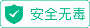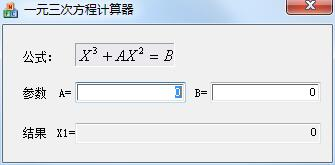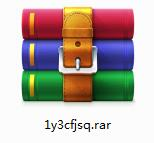# 一元三次方程计算器  1.0 官方版• 软件授权： 共享软件
• 软件大小： 0.6MB
• 软件评分：
• 软件类型： 国产软件
• 更新时间： 2022-01-13
• 应用平台： win7及以上
• 软件语言： 简体中文
• 版       本： 1.0 官方版方程定义

只含有一个未知数(即“元”)，并且未知数的最高次数为3(即“次”)的整式方程叫做一元三次方程(英文名：cubic equation of one unknown)。

本段方程标准

形如aX^3+bX^2+cX+d=0，(a，b，c，d∈R，且a≠0)的方程是一元三次方程的标准型。

X1=(Y1)^(1/3)+(Y2)^(1/3);

X2= (Y1)^(1/3)ω+(Y2)^(1/3)ω^2;

X3=(Y1)^(1/3)ω^2+(Y2)^(1/3)ω，

其中ω=(-1+i3^(1/2))/2;

Y(1,2)=-(q/2)±((q/2)^2+(p/3)^3)^(1/2)。

标准型一元三次方程aX ^3+bX ^2+cX+d=0，(a，b，c，d∈R，且a≠0)。

令X=Y—b/(3a)代入上式。

可化为适合卡尔丹公式直接求解的特殊型一元三次方程Y^3+pY+q=0。

1.在华军软件园下载一元三次方程计算器官方版的安装包2.下载后，将压缩包解压到以此软件命名的文件夹内3.打开文件夹，双击“exe”程序4.一元三次方程计算器为绿色软件，无需安装即可使用1.细节更出众!

2.BUG去无踪

## 网友评论华军网友华军网友华军网友

#### 本周最热软件本周最新软件